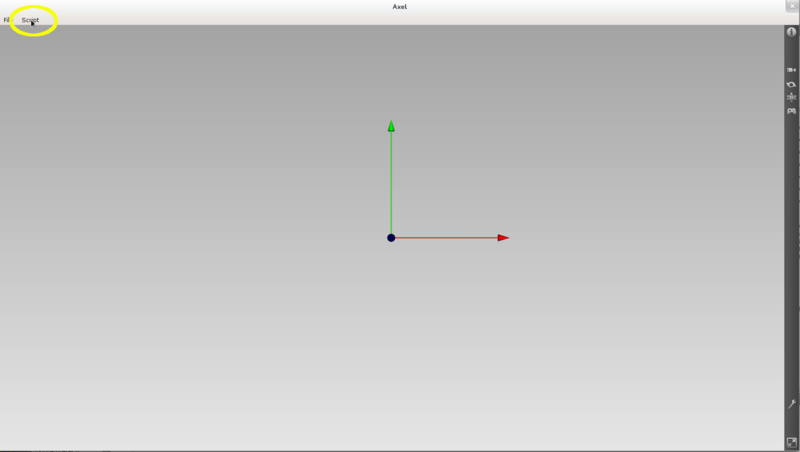# Using Python in Axl¶

`Python` interpreter is available in Axl from the git repository. You will find it in `Axl` distributions, for versions > 2.3.0.

## Open the `Python` script Interpreter in Axl¶

You can open the `Python` interpreter with the script menu in `Axl` tool bar:## Use the interpreter in console mode¶

Use the text editor as `Python` console. The text editor can interact in real time with `Axl` main window. It means that you can access to data or processes created in `Axl` windows or send new data to the view via the interpreter.

By pressing enter your send you current command to `Python` interpreter.

Let’s see some basic commands:

```a = 1+1    # compute basic addition in Python
print a    # will print 2
```

To the line without sending the command, you can end the current line by `:`. Then by pressing enter, you will just go back to the next line and continue your current command. To really send you command, you need to press enter twice.

Here is an example:

```tab = doubleArray(9)
for i in xrange(9):
tab[i] = 0.0
```

## Create and visualize axl data from Python¶

< - How to create an atomic data ?

In this example, we create a data of type axlPoint but it is similar for all atomic data of `Axl`:

```p1 = axlPoint(2.0, 0.1, 3)
```
• How can you add a data to the view ?

```axlView.add(p1)
```

`axlView` is a variable that allows you to acces the main view of `Axl`

• How to use a data type provided by a plugin ?

In this example, we use a bspline surface from the plugin BSplineTools`:

```s = newData("goDataSurfaceBSpline")
# creates a data from the data manager
```

`newData` is a helper function that will search inside the data factory if any axl plugin can create an `axlAbstractSurfaceBSpline` using the plugin bspline implementation.

• How to use a process provided by a plugin ?

In this example, we use the `offset` process from the plugin `BSplineTools`:

```p = newProcess("goProcessOffsetSurfaceBSpline")
# creates a process from the process manager
```

`newProcess` is a helper function that will search inside the process factory if any axl plugin can create a dtkAbstractProcess using the plugin offset process implementation.

## Save your commands as a Python script¶

All input commands are stored into a temporary buffer that you can use to write and save a Python script.

Let’s see an example that creates a point, a surface and applies an offset process. From an additionnal offset parameter and the bspline surface, the offset process computes and adds to the view the offset process output:

```n = axlPoint(1.0, 2.0, 3.0)

dtkSurface = newData("goDataSurfaceBSpline")
axlBSplineSurface = to_axl_surface_bspline(dtkSurface)

ku = doubleArray(9)
kv = doubleArray(9)
for i in xrange(4):
ku[i] = 0.0
kv[i] = 0.0

for j in xrange(5):
ku[j+4] = ku[j+3] + 1.0
kv[j+4] = kv[j+3] + 1.0

v= doubleArray(75)
for j in xrange(5):
for i in xrange(5):
v[3 * j * 5 + 3 * i ] = i
v[3 * j * 5 + 3 * i + 1] = j
v[3 * j * 5 + 3 * i + 2] = i*j

axlBSplineSurface.setSurface(5, 5, 4, 4, 3, ku, kv, v)

offsetProcess = newProcess("goProcessOffsetSurfaceBSpline")
offsetProcess.setInput(axlBSplineSurface, 0)
offsetProcess.setInput(n, 1)
distanceoffset = 1.0
offsetProcess.setParameter(distanceoffset)
offsetProcess.run()
offsetSurface = offsetProcess.output()
axlOffsetSurface = to_axl_data(offsetSurface)
axlOffsetSurface.setColor(1.0, 0.0, 0.0)
```

After typing this code in the console, you can use the save button of the interpreter to save your code as a Python script:

Open a Python script in `Axl`

You can also directly open a Python script using the button below:

A Python script can also be uploaded directly when running axl from a terminal:

```./axl  myscript.py
```

Basic geometric objects available from `Axl`. Here are some commands that you can use to create atomic data in `Axl`:

```print("Point" )
p1 = axlPoint()
print(p1)
print(" dir " )
print(dir(p1))
print(p1.description())

p2 = axlPoint(1.0, 2.0, 3.0)
p2.setColor (1.0, 0.0, 0.0)

p3 = axlPoint(0.0, 0.0, 5.0)

print("Line" )
line = axlLine(p1, p3)
print(line)
print(dir(line))
print(line.description())

print("Plane" )
n1 = axlPoint (0,1,0)
plane = axlPlane(p1, n1)
print(plane)
print(dir(plane))
print(plane.description())

print("Cone" )
cone = axlCone(p1, p2, 2.0)
print(cone)
print(dir(cone))
print(cone.description())

print("Cylinder" )
cylinder = axlCylinder(p1, p2, 1.0)
print(cylinder)
print(dir(cylinder))
print(cylinder.description())

print("Sphere" )
sphere = axlSphere(0, 5, 0, 2)
print(sphere)
print(dir(sphere))
print(sphere.description())

print("Ellipsoid" )
ellipsoid = axlEllipsoid()
print(ellipsoid)
print(dir(ellipsoid))
print(ellipsoid.description())